HOME COURSES PREVIEW REVIEW ABOUT CONTACTTOLL-FREE INFO & ORDERING: M-F: 9am-5pm (PST): (877) RAPID-10

 Quick Search: Keywords:

 Rapid Learning Member Area: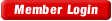Note: If you are a legacy user of chemistry24 members, please request a new login access to the premium server with your full name and old login email via vip@rapidlearningcenter.com

 Rapid Courses Catalog : Physics in 24 Hours Chemistry in 24 Hours Biology in 24 Hours Mathematics in 24 Hours

Weekly Physics Tips:
Want to become a top gun in your class? How about study less yet score high? Sign up this Physics Survival Weekly to learn how. Designed specifically for students who are taking physics, this free newsletter will show you how to survive and excel in class! Weekly topics include:
• How to Study Physics Effectively
• How to Read Physics Textbooks Easily
• How to Solve Physics Problems Systematically
• How to Score High on Physics Exams Strategically
• How to Master Physics Rapidly
Each week, you will receive study tips on the topics above and visual tutorial or study template to enhance your physics learning. Enter your name and email below to subscribe free:

 Physics Study Lounge These study sheets are for quick review on the subjects. Refer to our rapid courses for comprehensive review.     - Basic Skills in Physics     - Basic Math for Physics     - How to Solve Physics Problems     - Newton's Laws Study Guide     - ElectroMagnetism Quick Review     - Atomic Physics At-A-Glance     - Core Concepts in Nuclear Physics     - Special Relativity Overview

 Other Related Sites
 Note: For course links to launch, disable popup blockers or hold the ctrl key while clicking the link.

Special Relativity Overview

Key Physics Terms

• General relativity Theory dealing with how massive object bend or warp space and time around themselves.
• Special relativity Theory dealing with the effects of motion at near light speeds.
• Postulates of Special Relativity:  Two fundamental assumptions that form the basis of the theory of special relativity.
• Classical/Newtonian Physics:  Physics based upon Newton’s laws.  These laws work very well for motion that is not near the speed of light.  This physics is inaccurate for near light speed motion.
• Relativistic Physics:  Physics that describes the behavior of object that move at nearly the speed of light.  At low speeds, it simplifies to agree with Newtonian physics.
• Correspondence principle:  Any new theory must agree with an old theory when the old theory gives a correct result.
• Time dilation:  The slowing down of time in a frame of reference moving at relativistic speeds.
• Twin paradox:  An apparent contradiction where one twin takes a high speed trip and returns with a different age compared to the other twin.
• Muon:  A subatomic particle with a very short life span.  When moving at very high speeds, it seems to exist for a longer period of time.  This is one experimental piece of evidence that supports the theory of special relativity.
• Length contraction:  The shrinking of a distance or length due to motion at relativistic speeds.
• Rest energy:  The energy of mass itself, as given by the equation E=mc2
• Frame of Reference:  A viewpoint or set of coordinate axes to which position and motion can be described.

Key Formulas

• Time dilation:
•• Length contraction:
•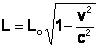• Relativistic momentum:
•• Total energy:
•• Rest energy:
•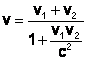•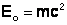Variables Used

• t= time
• to= original time, undilated time
• l=length
• lo=proper length, uncontracted length
• m=mass
• v=velocity, different velocities denoted as v1 and v2
• P=momentum
• c=speed of light, 3x108 m/s

1st Postulate of Special Relativity

• The laws of physics are the same in every inertial reference fram.
• No one fram of reference is better than any other.
• Any inertial reference frame is one where Newton’s law of inertia is valid.
• In a constantly moving bus, if you drop a penny, it will seem to obey the law of inertia, this is an inertial reference frame.
• Accelerating or rotating reference frames are not inertial.

2nd Postulate of Special Relativity

• The speed of light, measured in any inertial reference frame, always has the value of c, no matter how fast the source and/or observer are moving.
• A stationary observer sees the beam of light move at speed, c.
A moving source or observer also sees the beam of light move at speed c!
• This should seem to contradict common sense because our common everyday experiences are lacking any insight into motion at light speeds.

Typical Key Metric Units

• Time: seconds, s
• Length: meters, m
• Velocity: meters per second, m/s
• Momentum:  kilogram meters per second, kgm/s
• Mass:  kilograms, kg
• Energy:  Joules, J

Relativity Problem Solving Tips

• These tips will make it easier to solve any kinematics physics problems.
• Thoroughly read the entire problem.
• Draw a diagram if needed.
• Identify all given information.
• Identify the quantity to be found.
• Select appropriate formula(s) that incorporate what you know and what you want to find.
• Convert units if needed.  If all velocities are in term of c, you don’t need to convert to m/s.
• Be sure not to apply classical ideas to relativistic situations.

Typical Special Relativity Problem

Compare the classical and relativistic momentum of an electron moving at .99c.  The mass of an electron is given as   9.11x10-31kg.

Classical momentum:
P=mv
P=(9.11x10-31kg)(.99)(3x108m/s)
P=2.7x10-22kgm/s

Relativistic momentum: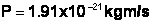Note how the high speed momentum is larger than the classical momentum.